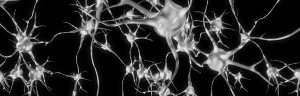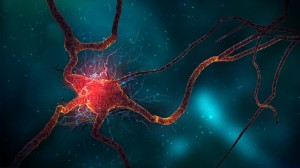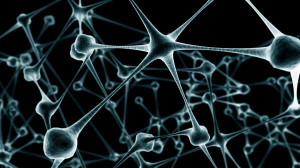# Category: Neural Networks

## Linguistic Regularities in Word Representations

In 2013, Mikolov et al. (2013) published a paper showing that complicated semantic analogy problems could be solved simply by adding and subtracting vectors learned with a neural network. Since then, there has been some more investigation into what is actually behind this method, and also some suggested improvements. This post is a summary/discussion of the paper “Linguistic Regularities in Sparse and Explicit Word Representations“, by Omer Levy and Yoav Goldberg, published at ACL 2014.

The task under consideration is analogy recovery. These are questions in the form:

a is to b as c is to d

In a usual setting, the system is given words a, b, c, and it needs to find d. For example:

‘apple’ is to ‘apples’ as ‘car’ is to ?

where the correct answer is ‘cars’. Or the well-known example:

‘man’ is to ‘woman’ as ‘king’ is to ?

where the desired answer is ‘queen’.

While methods such as relation extraction would also be completely reasonable approaches to this problem, the research is mainly focused on solving it by using vector similarity methods. This means we create vector representations for each of the words, and then use their positions in the high-dimensional feature space to determine what the missing word should be.

## Neural Networks, Part 3: The NetworkWe have learned about individual neurons in the previous section, now it’s time to put them together to form an actual neural network.

The idea is quite simple – we line multiple neurons up to form a layer, and connect the output of the first layer to the input of the next layer. Here is an illustration:

Each red circle in the diagram represents a neuron, and  the blue circles represent fixed values. From left to right, there are four columns: the input layer, two hidden layers, and an output layer. The output from neurons in the previous layer is directed into the input of each of the neurons in the next layer.

We have 3 features (vector space dimensions) in the input layer that we use for learning: $$x_1$$, $$x_2$$ and $$x_3$$. The first hidden layer has 3 neurons, the second one has 2 neurons, and the output layer has 2 output values. The size of these layers is up to you – on complex real-world problems we would use hundreds or thousands of neurons in each layer.

## Neural Networks, Part 2: The NeuronA neuron is a very basic classifier. It takes a number of input signals (a feature vector) and outputs a single value (a prediction). A neuron is also a basic building block of neural networks, and by combining together many neurons we can build systems that are capable of learning very complicated patterns. This is part 2 of an introductory series on neural networks. If you haven’t done so yet, you might want to start by learning about the background to neural networks in part 1.

Neurons in artificial neural networks are inspired by biological neurons in nervous systems (shown below). A biological neuron has three main parts: the main body (also known as the soma), dendrites and an axon. There are often many dendrites attached to a neuron body, but only one axon, which can be up to a meter long. In most cases (although there are exceptions), the neuron receives input signals from dendrites, and then outputs its own signals through the axon. Axons in turn connect to the dendrites of other neurons, using special connections called synapses, forming complex neural networks.

Below is an illustration of an artificial neuron, where the input is passed in from the left and the prediction comes out from the right. Each input position has a specific weight in the neuron, and they determine what output to give, given a specific input vector. For example, a neuron could be trained to detect cities. We can then take the vector for London from the previous section, give it as input to our neuron, and it will tell us it’s a city by outputting value 1. If we do the same for the word Tuesday, it will give a 0 instead, meaning that it’s not a city.